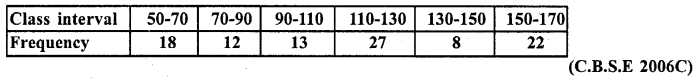• +91 9971497814
• info@interviewmaterial.com

# RD Chapter 7- Statistics Ex-7.3 Interview Questions Answers

### Related Subjects

Question 1 :
The following table gives the distribution of total household expenditure (in rupees) of manual workers in a city.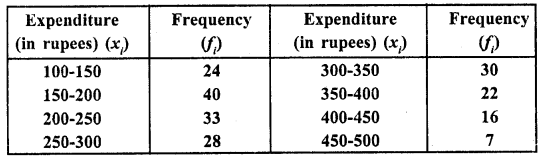Find the average expenditure ( in rupees ) per household.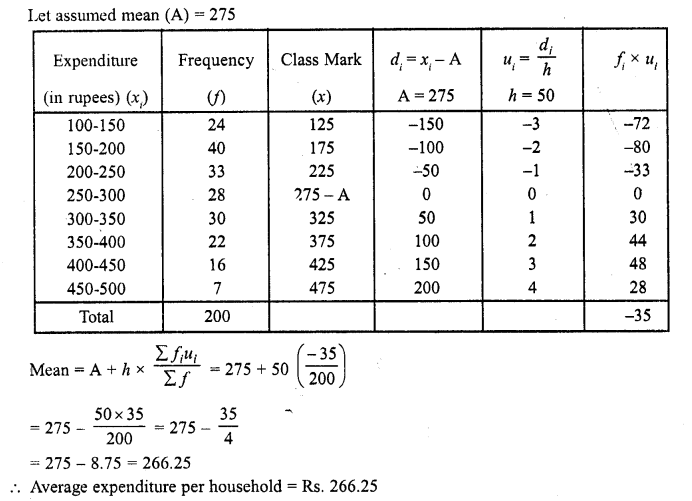Question 2 :
A survey was conducted by a group of students as a part of their environment awareness programme, in which they collected the following data regarding the number of plants in 20 houses in a locality. Find the mean number of plants per house.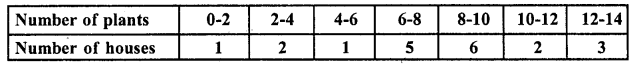Which method did you use for finding the mean, and why ?

Answer 2 : Let assumed mean (A) = 7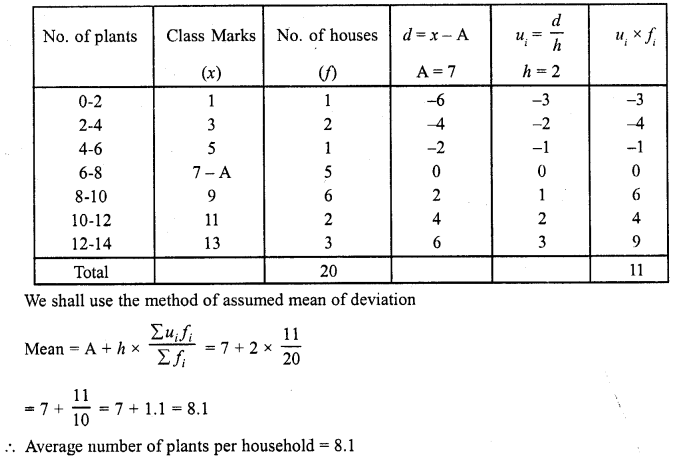Question 3 :
Consider the following distribution of daily wages of 50 workers of a facotry.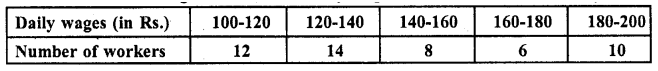Find the mean daily wages of the workers of the factory by using an appropriate method.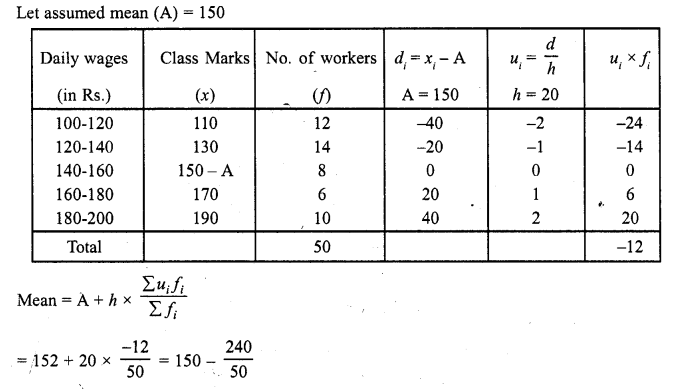= 150 – 4.80 = 145.20
Mean daily wages per worker = Rs. 145.20

Question 4 :
Thirty women were examined in a hospital by a doctor and the number of heart beats per minute recorded and summarised as follows. Find the mean heart beats per minute for these women, choosing a suitable method.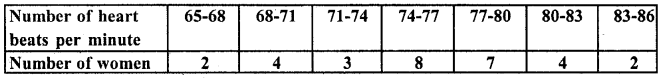Find the mean of each of the following frequency distribution (5 – 14)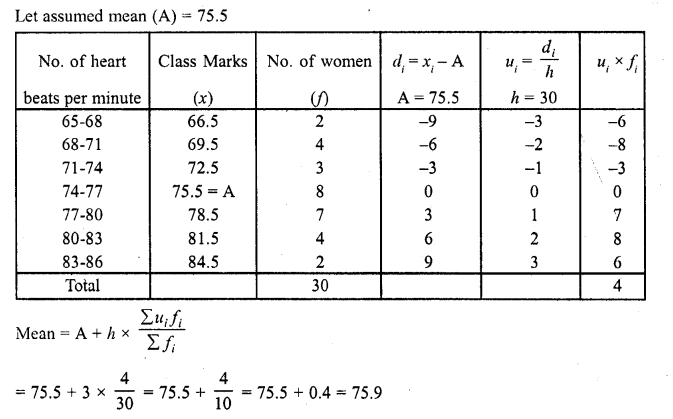Hence heart beats per minute = 75.9

Question 5 : Find the mean of each the following frequency distributions : (5 – 14)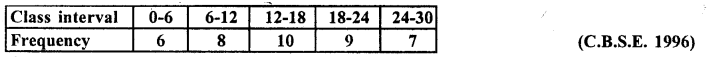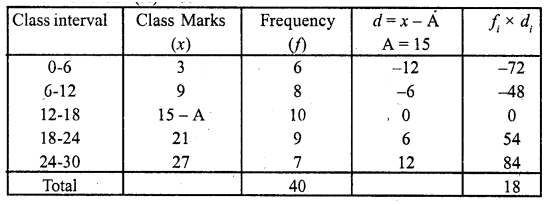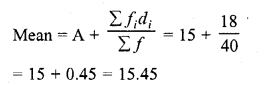Question 6 :